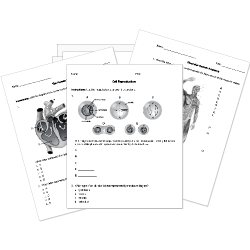Looking for Biology worksheets?
Check out our pre-made Biology worksheets!
 Tweet##### Browse Questions
• Arts (311)
• English Language Arts (5347)
• English as a Second Language ESL (3319)
• Health and Medicine (408)
• Life Skills (126)
• Math (1938)
• Physical Education (403)
• Science (6359)

• ### Solvents, Solutes, and Solubility

• #### Scientific Methods and Applications

• Social Studies (3813)
• Study Skills and Strategies (19)
• Technology (124)

You can create printable tests and worksheets from these Grade 8 Biochemical Pathways questions! Select one or more questions using the checkboxes above each question. Then click the add selected questions to a test button before moving to another page.

Previous Next
In what process do cells obtain energy from sugar molecules?
1. photosynthesis
2. nitrogen cycle
3. respiration
4. food chains
What are the products of photosynthesis?
1. water $(H_2O)$ and glucose $(C_6H_12O_6)$
2. glucose $(C_6H_12O_6)$ and carbon dioxide $(CO_2)$
3. carbon dioxide $(CO_2)$ and water $(H_2O)$
4. glucose $(C_6H_12O_6)$ and oxygen $(O_2)$
What are the reactants of cellular respiration?
1. water $(H_2O)$ and glucose $(C_6H_12O_6)$
2. glucose $(C_6H_12O_6)$ and carbon dioxide $(CO_2)$
3. carbon dioxide $(CO_2)$ and water $(H_2O)$
4. glucose $(C_6H_12O_6)$ and oxygen $(O_2)$
Which statement describes a primary distinction between respiration and photosynthesis?
1. Photosynthesis needs oxygen, while respiration needs water.
2. Photosynthesis needs carbon dioxide, while respiration needs oxygen and sugars.
3. Photosynthesis makes oxygen, while respiration makes water.
4. Photosynthesis makes water, while respiration makes carbon dioxide.
Which chemical process happens in cells to release energy?
1. respiration
2. photosynthesis
3. breathing
4. exercise
What process produces carbon dioxide?
1. photosynthesis
2. replication
3. mutation
4. respiration
Which of the following is TRUE statement about cellular respiration?
1. Cellular respiration converts energy directly from the Sun into energy stored in chemical bonds.
2. Cellular respiration cannot occur without sunlight.
3. Cellular respiration occurs in plant cells and in animal cells.
4. Cellular respiration is not a cycle with photosynthesis.
Which chemical reaction correctly summarizes photosynthesis?
1. $4 CO_2 + 6 H_2O + 2 O_2 = C_6H_12O_6$
2. $6 CO_2 + 6 H_2O + "energy" = C_6H_12O_6 + 6O_2$
3. $6 H_2O + 6 H_2 + "energy" = C_6H_12O_6$
4. $6 CO_2 + 6 H_2O + 6 O_2 = C_6H_12O_6 + O_2$
What does cellular respiration always release?
1. oxygen
2. carbon dioxide
3. energy
4. lactic acid
What is the source of energy for photosynthesis?
1. sunlight
2. carbon dioxide
3. glucose
4. water
Which statement is TRUE statement about cellular respiration?
1. Cellular respiration converts energy directly from the Sun into energy stored in chemical bonds.
2. Cellular respiration occurs in plant cells and in animal cells.
3. Cellular respiration cannot occur without sunlight.
4. None of the above
Plants make their own food through the process of                .
1. gametophytes
2. photokinesis
3. respiration
4. photosynthesis
What are the products of respiration?
1. water + carbon dioxide
2. oxygen + water
3. glucose + carbon dioxide
4. glucose + oxygen
Which is a product of photosynthesis?
1. carbon dioxide
2. light energy
3. oxygen
4. carbon monoxide
Which word equation represents the process of respiration?
1. oxygen + carbon dioxide -> water + glucose
2. water + carbon dioxide -> oxygen + glucose
3. carbon dioxide + glucose -> water + oxygen
4. oxygen + glucose -> water + carbon dioxide
Which word equation correctly represents photosynthesis?
1. carbon dioxide + oxygen -> glucose + water
2. carbon dioxide + water -> glucose + oxygen
3. oxygen + glucose -> carbon dioxide + water
4. water + oxygen -> glucose + carbon dioxide
What are the products of cellular respiration?
1. sugar, oxygen, and energy
2. carbon dioxide, water, and energy
3. oxygen, water, and energy
4. carbon dioxide, sugar, and energy